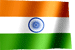RAMESH KRISHNA REDDYOnline Tutorials | PREV | TOP | NEXT

DRONA SERIES
COBOL STUDY MATERIAL

COBOL VERBS - DIVIDE

 ``` COBOL VERBS - DIVIDE DIVIDE Verb DIVIDE statement divide one number by another and store the result. Format 1. DIVIDE < literal-1 / data-item-1 > INTO data-item-1... Format 2. DIVIDE < literal-1 / data-item-1 > INTO < literal-2 / data-item-2 > GIVING data-item-3... REMAINDER data-item-4... Examples - (a) DIVIDE WS-A INTO WS-B. Above example is equals to WS-B = WS-B / WS-A. WS-B value is divided by WS-A and the result will be stored in WS-B. (b) DIVIDE WS-A INTO WS-B GIVING WS-C. Above example is equals to WS-C = WS-B / WS-A. WS-B value is divided by WS-A and the result will be stored in WS-C. No change to WS-B, WS-A values. (c) DIVIDE WS-A INTO WS-B GIVING WS-C REMAINDER WS-D. This statement very similar to example (b), except using REMAINDER word. after dividing WS-B by WS-A remainder value will be stored in WS-D. WS-A value is 100 WS-B value is 350 100 ) 350 ( 3 <--- This value will be moved to WS-C. 300 ---- 50 <--- Remainder, this value will be moved to WS-D. ---- Using BY instead of INTO.... we can use INTO instead of BY in the above format2. But, make sure that values/data-items used for calcualtion needs to be exchanged to get the same result. example (b) can be rewrite using word BY , to get same result in WS-C, need to swap WS-B, WS-A places. i.e, state should look like DIVIDE WS-B BY WS-A GIVING WS-C. equals WS-C = WS-B / WS-A. ```

NEXT CHAPTER TOPIC : COBOL

Visit COBOL books section in this site for good books